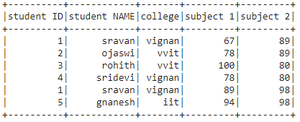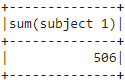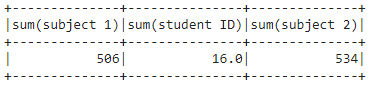Open in App
Not now

# How to find the sum of Particular Column in PySpark Dataframe

• Last Updated : 29 Jun, 2021

In this article, we are going to find the sum of PySpark dataframe column in Python. We are going to find the sum in a column using agg() function.

Let’s create a sample dataframe.

## Python3

 `# importing module``import` `pyspark`` ` `# importing sparksession from pyspark.sql module``from` `pyspark.sql ``import` `SparkSession`` ` `# creating sparksession and giving an app name``spark ``=` `SparkSession.builder.appName(``'sparkdf'``).getOrCreate()`` ` `# list  of students  data``data ``=` `[[``"1"``, ``"sravan"``, ``"vignan"``, ``67``, ``89``],``        ``[``"2"``, ``"ojaswi"``, ``"vvit"``, ``78``, ``89``],``        ``[``"3"``, ``"rohith"``, ``"vvit"``, ``100``, ``80``],``        ``[``"4"``, ``"sridevi"``, ``"vignan"``, ``78``, ``80``],``        ``[``"1"``, ``"sravan"``, ``"vignan"``, ``89``, ``98``],``        ``[``"5"``, ``"gnanesh"``, ``"iit"``, ``94``, ``98``]]`` ` `# specify column names``columns ``=` `[``'student ID'``, ``'student NAME'``, ``'college'``,``           ``'subject 1'``, ``'subject 2'``]`` ` `# creating a dataframe from the lists of data``dataframe ``=` `spark.createDataFrame(data, columns)`` ` `# display dataframe``dataframe.show()`

Output:### Using agg() method:

The agg() method returns the aggregate sum of the passed parameter column.

Syntax:

`dataframe.agg({'column_name': 'sum'})`

Where,

1. The dataframe is the input dataframe
2. The column_name is the column in the dataframe
3. The sum is the function to return the sum.

Example 1: Python program to find the sum in dataframe column

## Python3

 `# importing module``import` `pyspark`` ` `# importing sparksession from pyspark.sql module``from` `pyspark.sql ``import` `SparkSession`` ` `# creating sparksession and giving an app name``spark ``=` `SparkSession.builder.appName(``'sparkdf'``).getOrCreate()`` ` `# list  of students  data``data ``=` `[[``"1"``, ``"sravan"``, ``"vignan"``, ``67``, ``89``],``        ``[``"2"``, ``"ojaswi"``, ``"vvit"``, ``78``, ``89``],``        ``[``"3"``, ``"rohith"``, ``"vvit"``, ``100``, ``80``],``        ``[``"4"``, ``"sridevi"``, ``"vignan"``, ``78``, ``80``],``        ``[``"1"``, ``"sravan"``, ``"vignan"``, ``89``, ``98``],``        ``[``"5"``, ``"gnanesh"``, ``"iit"``, ``94``, ``98``]]`` ` `# specify column names``columns ``=` `[``'student ID'``, ``'student NAME'``, ``'college'``,``           ``'subject 1'``, ``'subject 2'``]`` ` `# creating a dataframe from the lists of data``dataframe ``=` `spark.createDataFrame(data, columns)`` ` ` ` `# find sum of subjects column``dataframe.agg({``'subject 1'``: ``'sum'``}).show()`

Output:Example 2: Get sum value from multiple columns

## Python3

 `# importing module``import` `pyspark`` ` `# importing sparksession from pyspark.sql module``from` `pyspark.sql ``import` `SparkSession`` ` `# creating sparksession and giving an app name``spark ``=` `SparkSession.builder.appName(``'sparkdf'``).getOrCreate()`` ` `# list  of students  data``data ``=` `[[``"1"``, ``"sravan"``, ``"vignan"``, ``67``, ``89``],``        ``[``"2"``, ``"ojaswi"``, ``"vvit"``, ``78``, ``89``],``        ``[``"3"``, ``"rohith"``, ``"vvit"``, ``100``, ``80``],``        ``[``"4"``, ``"sridevi"``, ``"vignan"``, ``78``, ``80``],``        ``[``"1"``, ``"sravan"``, ``"vignan"``, ``89``, ``98``],``        ``[``"5"``, ``"gnanesh"``, ``"iit"``, ``94``, ``98``]]`` ` `# specify column names``columns ``=` `[``'student ID'``, ``'student NAME'``, ``'college'``,``           ``'subject 1'``, ``'subject 2'``]`` ` `# creating a dataframe from the lists of data``dataframe ``=` `spark.createDataFrame(data, columns)`` ` ` ` `# find sum of multiple  column``dataframe.agg({``'subject 1'``: ``'sum'``, ``'student ID'``: ``'sum'``,``               ``'subject 2'``: ``'sum'``}).show()`

Output:My Personal Notes arrow_drop_up